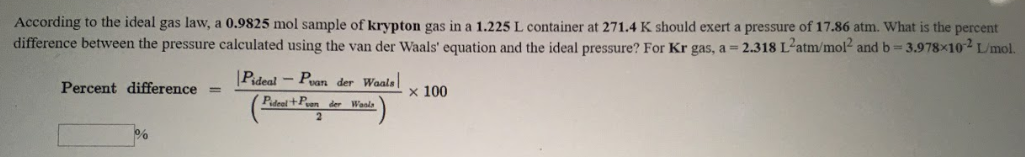# According to the ideal gas law, a 0.9825 mol sample of krypton gas is 1.225 L container at 271.4 K should exert a pressure of 17.86 atm. What is the percent difference between the pressure calculated using the van der Waal's equation and the ideal pressure? For Kr gas, a = 2.138 L^2atm/mol^2 and b = 3.978x10^-2 L/mol.